# Recursively divisible numbers

T. Fink

(2019)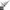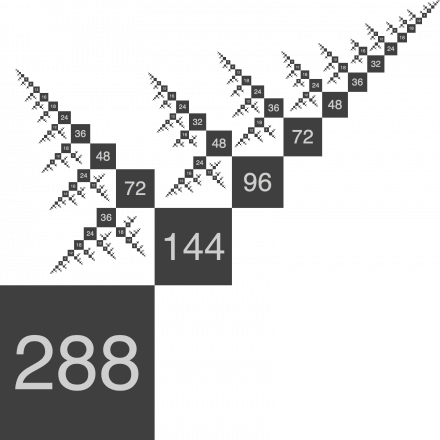Recursively divisible numbers are a new kind of number for designing modular systems across multiple organizational length scales.

Divisible numbers are useful whenever a whole needs to be divided into equal parts. But sometimes it is necessary to divide the parts into subparts, and subparts into sub-subparts, and so on, in a recursive way. To understand numbers that are recursively divisible, I introduce the recursive divisor function: a recursive analog of the usual divisor function. I give a geometric interpretation of recursively divisible numbers and study their general properties---in particular the number and sum of the recursive divisors. I show that the number of the recursive divisors is equal to twice the number of ordered factorizations into integers greater than one, a problem that has been much studied in its own right. By computing those numbers which are more recursively divisible than all of their predecessors, I recover many of the grid sizes commonly used in graphic design and digital technologies, and suggest new grid sizes which have not yet been adopted but should be. These are especially relevant to recursively modular systems which must operate across multiple organizational length scales.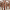#### Imaginary replica analysis of loopy regular random graphs

F. Lopez, T. Coolen

Sub. to Journal of Physics A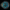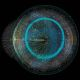#### Taming complexity

M. Reeves, S. Levin, T. Fink, A. Levina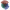#### The mathematical structure of innovation

T. Fink, I. Teimouri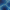#### Replica analysis of Bayesian data clustering

A. Mozeika,, A. Coolen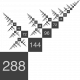#### Recursively divisible numbers

T. Fink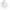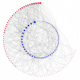#### Degree-correlations in a bursting dynamic network model

F. Vanni, P. Barucca

Journal of Economic Interaction and Coordination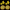#### Phase transition creates the geometry of the continuum from discrete space

R. Farr, T. Fink

Physical Review E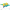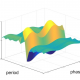#### Intelligently chosen interventions have potential to outperform the diode bridge in power conditioning

F. Liu, Y. Zhang, O. Dahlsten, F. Wang

Scientific Reports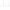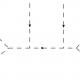#### Scale of non-locality for a system of n particles

S. Talaganis, I. Teimouri

Sub. to Physical Review D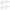#### How much can we influence the rate of innovation?

T. Fink, M. Reeves